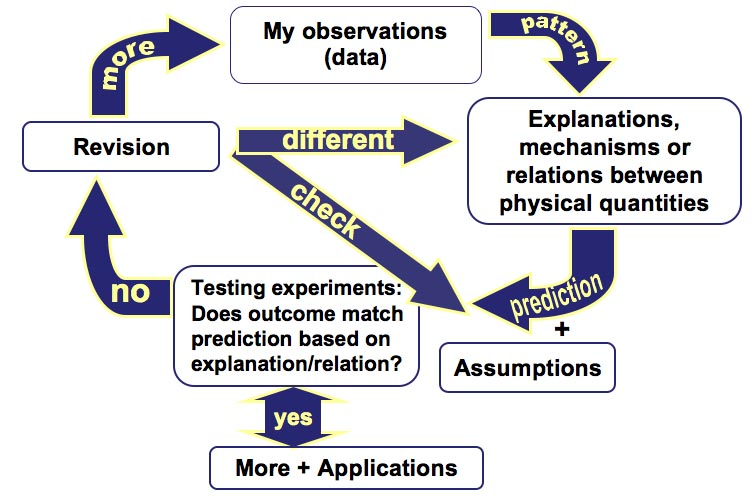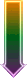## Introduction

#### Motivation:

Traditionally instructors use experiments in physical science instruction:

1. To illustrate a concept or a principle explained before
2. To verify a physical law taught in class. (For example: students conduct an experiment to verify Newton's second law.)

In both cases the instructor gives a lot of verbal and/or written guidance.

Research has shown that such use of experiments has been ineffective in helping acquire conceptual understanding or experimental skills.

#### The ISLE (Investigative Science Learning Environment) learning system and its philosophy:

Often students tend to learn physics as a group of disjointed concepts. They tend to accept scientific knowledge a "facts" without attempting to understand how it was constructed. But in every case in science, a person or people "invented" the laws as we know them though a complicated non-linear process. Very often students do not understand and are not encouraged to understand the coherent structure which underlies physics. We believe that it is the lack of this understanding which makes physical science traditionally "difficult" and decreases the level of confidence of the students.

If we look at scientific discovery through an historical lens, we can divide experiments into three broad categories. These are observational experiments (model/theory building experiments), testing experiments (model/theory testing experiments), or application experiments (application of the model/theory). These three categories of experiments joined together to construct a coherent scientific theory. The cycle may be summarized as follows:

1. A physicist observes a physical phenomenon or a series of related physical phenomena, collects data and uses available tools to find patterns.

2. She thinks of several possible qualitative explanations of the patterns and constructs analogies or different representations.

3. She devises experiments whose outcomes can be predicted using the above explanations and makes predictions of the outcomes of these experiments based on the explanation under test.

4. She conducts the experiments and compares the outcomes to the predictions. In case of a mismatch the explanation is revisited, in case of the match, now experiments are needed.

5. After the qualitative explanations fails to be disproved, a new cycle starts - a quantitative cycle. She may invent physical quantities to describe what is seen. She will look for quantitative patterns in the data.

6. If some interesting patterns are seen, she may propose several alternative models which fit the patterns. She might find mathematical relations between the physical quantities.

7. Different mathematical models may then be used to predict the results of new (unobserved) phenomena. Or a real world application may be devised.

8. She will then devise experiments to whose outcomes can be predicted using the quantitative models just devised or try out the application.

9. If the prediction of a particular model did not match the outcome of the experiment, one of possible reasons is that the model is flawed. However, a physicists should also consider other models (assumed to be correct) that were used to make a particular prediction before discarding the model. If the model is discarded, other models should be considered and tested in the same way as above. Alternatively, she may revise the initial model asking such questions as: What simplifying assumptions did I make? Is there a different model which would adequately describe the initial observations? Can I make a more accurate calculation? And so on. If the prediction of a model was verified or the application worked as expected, the physicist has gathered evidence that the model is successful in describing some physical phenomena.

The process can be summarized in the following diagram. Note that the cycle works for both qualitative and quantitative reasoningIn the spirit of a "cognitive apprenticeship" students learn the processes of science rather than the results. And the processes are learned by participation in them, ie: by observing and modelling the thought processes of an expert physicist, and by doing. Areas of physics are divided up into conceptual modules. Each module begins with one or more observational experiments. Little explanation is given, but scaffolding is provided to guide students through the technical details of making useful observations. Students examine the data, looking for patterns and relations between the physical quantities. The then construct a rule which models these patterns.

The observations and modelling will be followed by one or more testing/application experiments. Students either use their model to make a prediction and then devise an experiment to test the prediction, or they are given a challenge which requires them to use their model to predict an outcome of a specific experiment. If things do not work out as planned, students have to go back and revise their model, the assumptions made, the accuracy of any calculations and so on.

In the testing/application stage, the following reasoning scheme can be observed and practiced so that students acquire the skills of what is called "hypothetico-deductive reasoning".

# Before Experiment is Performed

## IF

Explanation/rule/idea is reasonable

AND we assume...

Hypothesis or proposed model## AND

We do x

Suggested testing experiment## THEN

We would expect y to happen

# After experiment has been performed

Expected outcome occurred

## ← AND|BUT →

Expected outcome did NOT occurExplanation/rule/idea has not yet been disproven

## ←ĀĀĀĀĀĀĀ→

EITHER: We made a mistake with our assumptions etc... OR Explanation/rule/idea is not valid in this regime

In following this process through, students are engaging in a process of mental modelling, constructing their own knowledge of physics in the same way as physicists do it. Not only do they understand the physics they are doing, but they are equipped with learning skills useful in any branch of science.

#### How to use this web page

The web page provides examples of experiments that can be used in class or for homework for the students to observe physical phenomena, collect data, analyze data, build models and test their predictions. Students should not make any predictions before viewing observational experiments, the purpose of these experiments is to help students accumulate enough experimental data to make predictions based on some knowledge. Prediction experiments require predictions based on the explanations (models) that students proposed to explain the observational experiments. It is better to elicit several alternative models and then view the experiment. See example of a prediction .

The write-ups and videos are provided in a logical sequence. It is sometimes helpful to download the videos and analyze them through quicktime itself rather than through the web page since the videos can be enlarged and examined at greater detail. Each video clip can be viewed in real time if a viewer presses the "play" button and in slow motion, frame by frame, if the viewer presses the cursor keys once per each frame. Click for an example. Each clip is digitized at a different number of frames per second, please notice for each clip how many fps were used. There are no built in measuring instruments in the clips. The viewer has to make a conscious decision on what she/he wants to measure. The viewer must decide how to collect data, how to store it and how it should be analyzed. Example . Data analysis can be done using Excel. Position data can be collected using the rulers in the videos or using transperency paper attached to the computer screen.

#### References

1. E. Etkina & A. Van Heuvelen, "Investigative Science Learning Environment--A Science Process Approach to Learning Physics" in Research-Based Reform of University Physics edited by E. F. Redish and P. J. Cooney, 2007.
2. E. Etkina, A. Van Heuvelen, S. White-Brahmia, D. T. Brookes, M. Gentile, S. Murthy, D. Rosengrant, & A. Warren, "Scientific abilities and their assessment," Phys. Rev. ST Phys. Educ. Res. 2, 020103, 2006.
3. E. Etkina, S. Murthy, & X. Zou, "Using introductory labs to engage students in experimental design," Am. J. Phys. 74, 979-986, 2006.
4. E. Etkina, A. Karelina, & M. Ruibal-Villasenor, "How long does it take? A study of student acquisition of scientific abilities," Phys. Rev. ST Phys. Educ. Res. 4, 020108, 2006.